# Catch up on the latest AI articles# Improved Generated Samples Using Gradient Flow [ICLR2021]

3 main points
✔️ Proposal of a method to improve the quality of samples produced by deep generative models (DG$f$low )
✔️ Extension of DG$f$low to VAEs and normalized flows, which are generative models that explicitly handle likelihoods
✔️ Confirmed the improvement of the quality of the generated samples on image and text datasets

written by Abdul Fatir AnsariMing Liang AngHarold Soh
(Submitted on 1 Dec 2020 (v1), last revised 5 Jun 2021 (this version, v4))

Subjects: Machine Learning (cs.LG); Artificial Intelligence (cs.AI); Machine Learning (stat.ML)code：The images used in this article are from the paper or created based on it.

## first of all

Deep generative models are an area of the recent development in machine learning. The study of deep generative models aims to artificially generate data that is very similar to data that exists in the real world.

GAN (Generative Adversarial Networks) is one of the deep learning models, which consists of two kinds of neural networks, a Discriminator to discriminate real data and generated data, and Generator to generate samples. GANs are trained by minimizing the "distance" between the real data distribution and the generated data distribution by minimax optimization.

Since the purpose of GAN is to generate new data that is very similar to real data, it was common practice to discard the Discriminator and generate samples using only the Generator once training was complete.

In this article, we introduce a framework (DG$f$low) to improve poor quality generated samples using the information about the real data distribution left in the trained Discriminator.

Before going into the concrete explanation of DG$F$low, I will explain the gradient flow. The gradient flow is the "shortest path" in the process of minimizing the scalar function $F(x)$.

Here, "shortest path" means that the movement at each time is oriented in the direction that minimizes $F$. Thus the gradient flow $\mathbf{x}(t)$ satisfies the following equation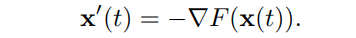## Improved generated samples using gradient flow (DG$f$low)

One of the problems with conventional deep generative models is that the quality of the generated data differs greatly depending on the samples in the latent space. To improve the performance of the generative model, it is important to know how to reduce the amount of poor quality data. However, in DG$f$low, we propose a method to improve the performance without discarding poor quality samples.

Consider $F$, which we want to minimize as the first step in constructing the gradient flow. This is not much different from the loss function in conventional GAN, which is the $F$-divergence representing the "distance" between the generated and real data distributions. However, a negative entropy term is added to ensure diversity when simulating gradient flows at discrete time steps. The function $F$ is defined as follows where $\mu$ is the probability measure of the real data and $\rho$ is the probability measure of the generated data.As a next step, we consider the gradient flow of $F$. This gradient flow can be expressed as the Fokker-Plank equation, which is a kind of partial differential equation, and it is known that $\mathbf{x}$ satisfying this equation follows the McKean-Vlasov process, which is a kind of stochastic process. The data point $\mathbf{x_t}$ at each point in time can be obtained by performing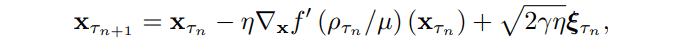### From improvement in data space to improvement in latent space

The numerical simulation in (1) shows that the sample improvement procedure is performed in the data space, but in the case of high-dimensional data such as images, errors accumulate and the quality of the generated data becomes poor. Although the condition of the generator is not always satisfied, it has been empirically shown to work well.

Based on the above, the algorithm for DG$f$low is as follows.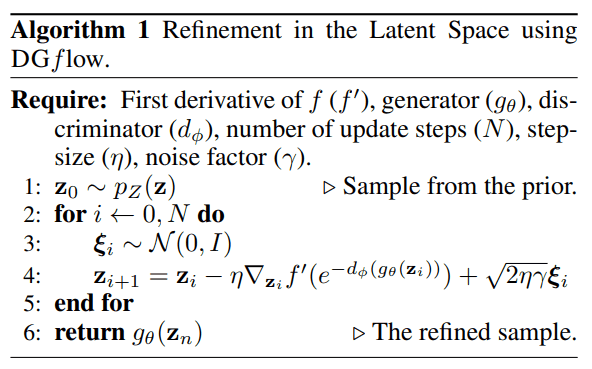## experimental results

### Validation on two-dimensional data sets

First, we checked the performance of DG$f$low on a two-dimensional artificial dataset (25Gaussians [top], 2DSwissroll [bottom]). For each dataset, we train WGAN-GP (blue) and then improve the samples with three different methods (red), including DG$f$low.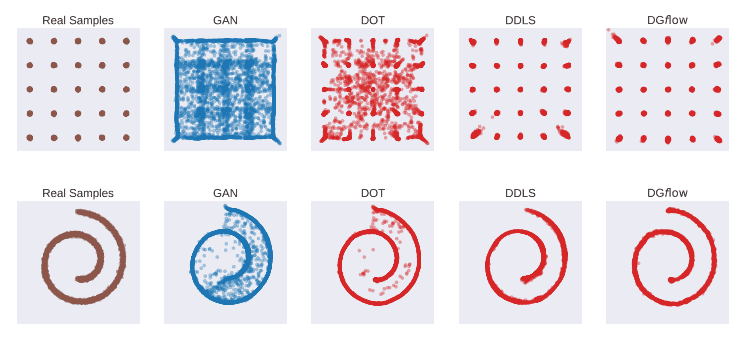From the above figure, we can see that some of the samples generated by WGAN-GP are far from the real data, and DG$f$low and DDLS can improve them.

### Validation of image data sets

For image data generation, we used the CIFAR10 and STL10 data sets. Two metrics, Frechet Inception Distance (FID) and Inception Score (IS) were used to evaluate the generated samples. (In this blog, we only deal with the FID comparison.) The smaller the value of FID, the better the index.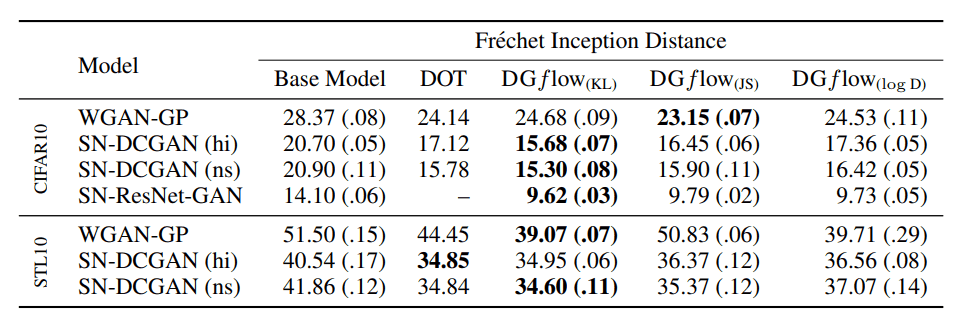In the table above, the FIDs are compared. WGAN-GP, SN-DCGAN, and SN-ResNet-GAN are used as the deep generative models as the base. These are all GANs where the Discriminator outputs a scalar. In most cases, the performance of our method outperforms that of the conventional method, DOT.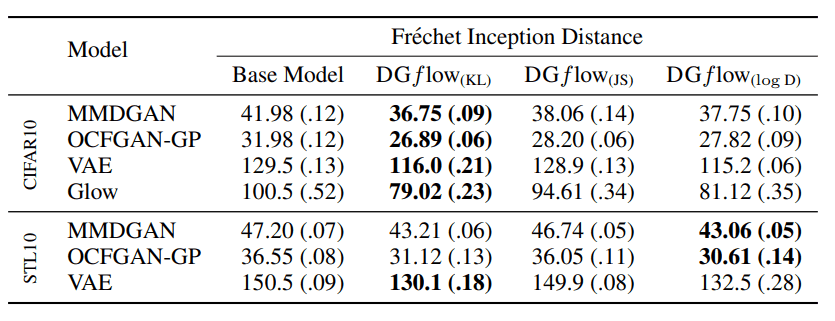The table above shows the results of testing various types of deep generative models as a base. For example, MMDGAN is a derivative of GAN in which the output of the Discriminator is a vector. Also, VAE and Glow are deep generative models that explicitly handle log-likelihood, which is different from GAN. It was shown that DGflow can be used to improve the samples even when the architectures and generative models are different.

### Validation of linguistic data sets

For text data generation, we used the Billion Words Dataset, which is used for character-level language modeling; the Billion Words Dataset is a preprocessed dataset of 32 character strings. To evaluate the generated samples, we use the JS divergence calculated between the generated samples and the real data n-grams. (JS-4, JS-6)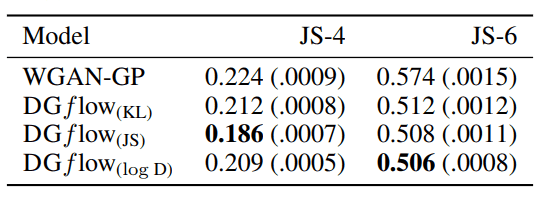From the above table, we can see that the samples generated by WGAN-GP are also improved by DG$f$low for text data.

## in the end

What do you think? DG$f$low is a powerful framework that raises the quality of the generated samples regardless of the type of deep generative model (GAN, VAE, normalized flow). However, the number of time steps in the simulation of gradient flows is a hyperparameter, and how to determine it may be a matter of debate. Interestingly, the name of the method is a combination of "$f$" of $f$-divergence and "flow" of gradient flow.

If you're interested in the theoretical background on the numerical simulation of gradient flows, please read the original paper!

If you have any suggestions for improvement of the content of the article,